Hostname: page-component-546b4f848f-bvkm5 Total loading time: 0 Render date: 2023-06-03T11:08:24.869Z Has data issue: false Feature Flags: { "useRatesEcommerce": true } hasContentIssue false

# The unifying theory of scaling in thermal convection: the updated prefactors

Published online by Cambridge University Press:  30 July 2013

*
Email addresses for correspondence: r.j.a.m.stevens@utwente.nl, d.lohse@utwente.nl
Email addresses for correspondence: r.j.a.m.stevens@utwente.nl, d.lohse@utwente.nl

## Abstract

The unifying theory of scaling in thermal convection (Grossmann & Lohse, J. Fluid. Mech., vol. 407, 2000, pp. 27–56; henceforth the GL theory) suggests that there are no pure power laws for the Nusselt and Reynolds numbers as function of the Rayleigh and Prandtl numbers in the experimentally accessible parameter regime. In Grossmann & Lohse (Phys. Rev. Lett., vol. 86, 2001, pp. 3316–3319) the dimensionless parameters of the theory were fitted to 155 experimental data points by Ahlers & Xu (Phys. Rev. Lett., vol. 86, 2001, pp. 3320–3323) in the regime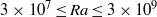$3\times 1{0}^{7} \leq \mathit{Ra}\leq 3\times 1{0}^{9}$ and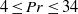$4\leq \mathit{Pr}\leq 34$ and Grossmann & Lohse (Phys. Rev. E, vol. 66, 2002, p. 016305) used the experimental data point from Qiu & Tong (Phys. Rev. E, vol. 64, 2001, p. 036304) and the fact that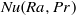$\mathit{Nu}(\mathit{Ra}, \mathit{Pr})$ is independent of the parameter$a$, which relates the dimensionless kinetic boundary thickness with the square root of the wind Reynolds number, to fix the Reynolds number dependence. Meanwhile the theory is, on the one hand, well-confirmed through various new experiments and numerical simulations; on the other hand, these new data points provide the basis for an updated fit in a much larger parameter space. Here we pick four well-established (and sufficiently distant)$\mathit{Nu}(\mathit{Ra}, \mathit{Pr})$ data points and show that the resulting$\mathit{Nu}(\mathit{Ra}, \mathit{Pr})$ function is in agreement with almost all established experimental and numerical data up to the ultimate regime of thermal convection, whose onset also follows from the theory. One extra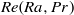$\mathit{Re}(\mathit{Ra}, \mathit{Pr})$ data point is used to fix$\mathit{Re}(\mathit{Ra}, \mathit{Pr})$. As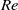$\mathit{Re}$ can depend on the definition and the aspect ratio, the transformation properties of the GL equations are discussed in order to show how the GL coefficients can easily be adapted to new Reynolds number data while keeping$\mathit{Nu}(\mathit{Ra}, \mathit{Pr})$ unchanged.

## JFM classification

Type
Papers
Information
Journal of Fluid Mechanics , 10 September 2013 , pp. 295 - 308

## Access options

Get access to the full version of this content by using one of the access options below. (Log in options will check for institutional or personal access. Content may require purchase if you do not have access.)

## References

Ahlers, G. 2000 Effect of sidewall conductance on heat-transport measurements for turbulent Rayleigh–Bénard convection. Phys. Rev. E 63, 015303.CrossRefGoogle ScholarPubMed
Ahlers, G., Bodenschatz, E., Funfschilling, D., Grossmann, S., He, X., Lohse, D., Stevens, R. J. A. M. & Verzicco, R. 2012a Logarithmic temperature profiles in turbulent Rayleigh–Bénard convection. Phys. Rev. Lett. 109, 114501.CrossRefGoogle ScholarPubMed
Ahlers, G., Bodenschatz, E., Funfschilling, D. & Hogg, J. 2009a Turbulent Rayleigh–Bénard convection for a Prandtl number of 0.67. J. Fluid Mech. 641, 157167.CrossRefGoogle Scholar
Ahlers, G., Grossmann, S. & Lohse, D. 2009b Heat transfer and large-scale dynamics in turbulent Rayleigh–Bénard convection. Rev. Mod. Phys. 81, 503537.CrossRefGoogle Scholar
Ahlers, G., He, X., Funfschilling, D. & Bodenschatz, E. 2012b Heat transport by turbulent Rayleigh–Bénard convection for$\mathit{Pr}= 0. 8$ and$3\times 1{0}^{12} \leq \mathit{Ra}\leq 1{0}^{15}$ : aspect ratio$\Gamma = 0. 50$ . New J. Phys. 14, 063030.Google Scholar
Ahlers, G. & Xu, X. 2001 Prandtl-number dependence of heat transport in turbulent Rayleigh–Bénard convection. Phys. Rev. Lett. 86, 33203323.CrossRefGoogle ScholarPubMed
Breuer, M., Wessling, S., Schmalzl, J. & Hansen, U. 2004 Effect of inertia in Rayleigh–Bénard convection. Phys. Rev. E 69, 026302.CrossRefGoogle ScholarPubMed
Brown, E., Funfschilling, D., Nikolaenko, A. & Ahlers, G. 2005 Heat transport by turbulent Rayleigh–Bénard convection: effect of finite top- and bottom conductivity. Phys. Fluids 17, 075108.CrossRefGoogle Scholar
Burnishev, Y., Segre, E. & Steinberg, V. 2010 Strong symmetrical non-Oberbeck–Boussinesq turbulent convection and the role of compressibility. Phys. Fluids 22, 035108.CrossRefGoogle Scholar
Castaing, B., Gunaratne, G., Heslot, F., Kadanoff, L., Libchaber, A., Thomae, S., Wu, X. Z., Zaleski, S. & Zanetti, G. 1989 Scaling of hard thermal turbulence in Rayleigh–Bénard convection. J. Fluid Mech. 204, 130.CrossRefGoogle Scholar
Chaumat, S., Castaing, B. & Chilla, F. 2002 Rayleigh–Bénard cells: influence of plate properties. In Advances in Turbulence IX (ed. Castro, I. P., Hancock, P. E. & Thomas, T. G.). International Center for Numerical Methods in Engineering, CIMNE.Google Scholar
Chavanne, X., Chilla, F., Castaing, B., Hebral, B., Chabaud, B. & Chaussy, J. 1997 Observation of the ultimate regime in Rayleigh–Bénard convection. Phys. Rev. Lett. 79, 36483651.CrossRefGoogle Scholar
Chavanne, X., Chilla, F., Chabaud, B., Castaing, B. & Hebral, B. 2001 Turbulent Rayleigh–Bénard convection in gaseous and liquid He. Phys. Fluids 13, 13001320.CrossRefGoogle Scholar
Cioni, S., Ciliberto, S. & Sommeria, J. 1997 Strongly turbulent Rayleigh–Bénard convection in mercury: comparison with results at moderate Prandtl number. J. Fluid Mech. 335, 111140.CrossRefGoogle Scholar
Emran, M. S. & Schumacher, J. 2012 Conditional statistics of thermal dissipation rate in turbulent Rayleigh–Bénard convection. Eur. Phys. J. E 108, 3542.Google Scholar
Fleischer, A. S. & Goldstein, R. J. 2002 High-Rayleigh-number convection of pressurized gases in a horizontal enclosure. J. Fluid Mech. 469, 112.CrossRefGoogle Scholar
Funfschilling, D., Brown, E., Nikolaenko, A. & Ahlers, G. 2005 Heat transport by turbulent Rayleigh–Bénard convection in cylindrical cells with aspect ratio one and larger. J. Fluid Mech. 536, 145154.CrossRefGoogle Scholar
Glazier, J. A., Segawa, T., Naert, A. & Sano, M. 1999 Evidence against ultrahard thermal turbulence at very high Rayleigh numbers. Nature 398, 307310.CrossRefGoogle Scholar
Grossmann, S. & Lohse, D. 2000 Scaling in thermal convection: a unifying view. J. Fluid. Mech. 407, 2756.CrossRefGoogle Scholar
Grossmann, S. & Lohse, D. 2001 Thermal convection for large Prandtl number. Phys. Rev. Lett. 86, 33163319.CrossRefGoogle Scholar
Grossmann, S. & Lohse, D. 2002 Prandtl and Rayleigh number dependence of the Reynolds number in turbulent thermal convection. Phys. Rev. E 66, 016305.CrossRefGoogle ScholarPubMed
Grossmann, S. & Lohse, D. 2004 Fluctuations in turbulent Rayleigh–Bénard convection: the role of plumes. Phys. Fluids 16, 44624472.CrossRefGoogle Scholar
Grossmann, S. & Lohse, D. 2011 Multiple scaling in the ultimate regime of thermal convection. Phys. Fluids 23, 045108.CrossRefGoogle Scholar
He, X., Funfschilling, D., Bodenschatz, E. & Ahlers, G. 2012a Heat transport by turbulent Rayleigh–Bénard convection for$\Pr = 0. 8$ and$4\times 1{0}^{11} \leq \mathit{Ra}\leq 2\times 1{0}^{14}$ for aspect ratio$\Gamma = 1. 00$ . New J. Phys. 14, 103012.Google Scholar
He, X., Funfschilling, D., Nobach, H., Bodenschatz, E. & Ahlers, G. 2012b Transition to the ultimate state of turbulent Rayleigh–Bénard convection. Phys. Rev. Lett. 108, 024502.CrossRefGoogle ScholarPubMed
He, X., He, G. & Tong, P. 2010 Small-scale turbulent fluctuations beyond Taylor’s frozen-flow hypothesis. Phys. Rev. E 81, 065303.CrossRefGoogle ScholarPubMed
He, X. & Tong, P. 2011 Kraichnan’s random sweeping hypothesis in homogeneous turbulent convection. Phys. Rev. E 83, 037302.CrossRefGoogle ScholarPubMed
He, G. W. & Zhang, J. B. 2006 Elliptic model for space–time correlations in turbulent shear flows. Phys. Rev. E 73, 055303.CrossRefGoogle ScholarPubMed
Horanyi, S., Krebs, L. & Müller, U. 1999 Turbulent Rayleigh–Bénard convection in low Prandtl number fluids. Intl J. Heat Mass Transfer 42, 39834003.CrossRefGoogle Scholar
Kerr, R. & Herring, J. R. 2000 Prandtl number dependence of Nusselt number in direct numerical simulations. J. Fluid Mech. 419, 325344.CrossRefGoogle Scholar
Lakkaraju, R., Stevens, R. J. A. M., Verzicco, R., Grossmann, S., Prosperetti, A., Sun, C. & Lohse, D. 2012 Spatial distribution of heat flux and fluctuations in turbulent Rayleigh–Bénard convection. Phys. Rev. E 86, 056315.CrossRefGoogle Scholar
Landau, L. D. & Lifshitz, E. M. 1987 Fluid Mechanics. Pergamon.Google Scholar
Lohse, D. & Xia, K. Q. 2010 Small-scale properties of turbulent Rayleigh–Bénard convection. Annu. Rev. Fluid Mech. 42, 335364.CrossRefGoogle Scholar
Niemela, J., Skrbek, L., Sreenivasan, K. R. & Donnelly, R. 2000 Turbulent convection at very high Rayleigh numbers. Nature 404, 837840.CrossRefGoogle ScholarPubMed
Niemela, J., Skrbek, L., Sreenivasan, K. R. & Donnelly, R. J. 2001 The wind in confined thermal turbulence. J. Fluid Mech. 449, 169178.CrossRefGoogle Scholar
Niemela, J. & Sreenivasan, K. R. 2003 Confined turbulent convection. J. Fluid Mech. 481, 355384.CrossRefGoogle Scholar
Niemela, J. & Sreenivasan, K. R. 2006 Turbulent convection at high Rayleigh numbers and aspect ratio 4. J. Fluid Mech. 557, 411422.CrossRefGoogle Scholar
Petschel, K., Stellmach, S., Wilczek, M., Lülff, J. & Hansen, U. 2013 Dissipation layers in Rayleigh–Bénard convection: a unifying view. Phys. Rev. Lett. 110, 114502.CrossRefGoogle ScholarPubMed
Qiu, X. L. & Tong, P. 2001 Large scale velocity structures in turbulent thermal convection. Phys. Rev. E 64, 036304.CrossRefGoogle ScholarPubMed
Roche, P. E., Castaing, B., Chabaud, B., Hebral, B. & Sommeria, J. 2001 Side wall effects in Rayleigh–Bénard experiments. Eur. Phys. J. B 24, 405408.CrossRefGoogle Scholar
Roche, P.-E., Gauthier, F., Kaiser, R. & Salort, J. 2010 On the triggering of the ultimate regime of convection. New J. Phys. 12, 085014.CrossRefGoogle Scholar
Rossby, H. T. 1969 A study of Bénard convection with and without rotation. J. Fluid Mech. 36, 309335.CrossRefGoogle Scholar
Schlichting, H. 1979 Boundary Layer Theory, 7th edn. McGraw-Hill.Google Scholar
Shishkina, O., Stevens, R. J. A. M., Grossmann, S. & Lohse, D. 2010 Boundary layer structure in turbulent thermal convection and its consequences for the required numerical resolution. New J. Phys. 12, 075022.CrossRefGoogle Scholar
Shishkina, O. & Thess, A. 2009 Mean temperature profiles in turbulent Rayleigh–Bénard convection of water. J. Fluid Mech. 633, 449460.CrossRefGoogle Scholar
Siggia, E. D. 1994 High Rayleigh number convection. Annu. Rev. Fluid Mech. 26, 137168.CrossRefGoogle Scholar
Stevens, R. J. A. M., Clercx, H. J. H. & Lohse, D. 2010a Boundary layers in rotating weakly turbulent Rayleigh–Bénard convection. Phys. Fluids 22, 085103.CrossRefGoogle Scholar
Stevens, R. J. A. M., Clercx, H. J. H. & Lohse, D. 2010b Optimal Prandtl number for heat transfer in rotating Rayleigh–Bénard convection. New J. Phys. 12, 075005.CrossRefGoogle Scholar
Stevens, R. J. A. M., Lohse, D. & Verzicco, R. 2011a Prandtl number dependence of heat transport in high Rayleigh number thermal convection. J. Fluid. Mech. 688, 3143.CrossRefGoogle Scholar
Stevens, R. J. A. M., Overkamp, J., Lohse, D. & Clercx, H. J. H. 2011b Effect of aspect-ratio on vortex distribution and heat transfer in rotating Rayleigh–Bénard convection. Phys. Rev. E 84, 056313.CrossRefGoogle ScholarPubMed
Stevens, R. J. A. M., Verzicco, R. & Lohse, D. 2010c Radial boundary layer structure and Nusselt number in Rayleigh–Bénard convection. J. Fluid. Mech. 643, 495507.CrossRefGoogle Scholar
Sun, C., Ren, L.-Y., Song, H. & Xia, K.-Q. 2005 Heat transport by turbulent Rayleigh–Bénard convection in 1m diameter cylindrical cells of widely varying aspect ratio. J. Fluid Mech. 542, 165174.CrossRefGoogle Scholar
Sun, C. & Xia, K.-Q. 2005 Scaling of the Reynolds number in turbulent thermal convection. Phys. Rev. E 72, 067302.CrossRefGoogle ScholarPubMed
Urban, P., Hanzelka, P., Kralik, T., Musilova, V., Srnka, A. & Skrbek, L. 2012 Effect of boundary layers asymmetry on heat transfer efficiency in turbulent Rayleigh–Bénard convection at very high Rayleigh numbers. Phys. Rev. Lett. 109, 154301.CrossRefGoogle ScholarPubMed
Urban, P., Musilová, V. & Skrbek, L. 2011 Efficiency of heat transfer in turbulent Rayleigh–Bénard convection. Phys. Rev. Lett. 107, 014302.CrossRefGoogle ScholarPubMed
van der Poel, E. P., Stevens, R. J. A. M. & Lohse, D. 2013 Comparison between two and three-dimensional Rayleigh–Bénard convection. J. Fluid Mech. (Submitted).Google Scholar
Verzicco, R. 2002 Sidewall finite conductivity effects in confined turbulent thermal convection. J. Fluid Mech. 473, 201210.CrossRefGoogle Scholar
Verzicco, R. & Camussi, R. 1999 Prandtl number effects in convective turbulence. J. Fluid Mech. 383, 5573.CrossRefGoogle Scholar
Xia, K.-Q., Lam, S. & Zhou, S. Q. 2002 Heat-flux measurement in high-Prandtl-number turbulent Rayleigh–Bénard convection. Phys. Rev. Lett. 88, 064501.CrossRefGoogle ScholarPubMed
Zhao, X. & He, G.-W. 2009 Space–time correlations of fluctuating velocities in turbulent shear flows. Phys. Rev. E 79, 046316.CrossRefGoogle ScholarPubMed
Zhou, Q., Li, C.-M., Lu, Z.-M. & Liu, Y.-L. 2011 Experimental investigation of longitudinal space–time correlations of the velocity field in turbulent Rayleigh–Bénard convection. J. Fluid Mech. 683, 94111.CrossRefGoogle Scholar